# Precalculus : Solve and Graph Rational Inequalities

## Example Questions

### Example Question #1 : Solve And Graph Rational Inequalities

Solve the inequality.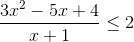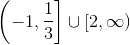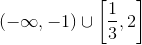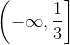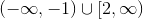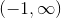Explanation:

First, subtractfrom both sides so you get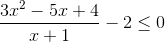.

Then find the common denominator and simplify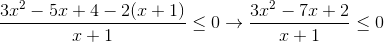.

Next, factor out the numerator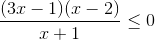and set each of the three factor equal to zero and solve for.

The solutions are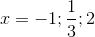.

Now plug in values between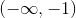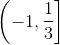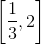, and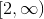into the inequality and observe if the conditions of the inequality are met.

Note that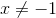. They are met in the intervaland.

Thus, the solution to the inequality is### Example Question #1 : Solve And Graph Rational Inequalities

Solve and graph: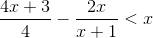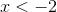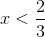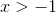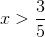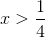Explanation:

1) Multiply both sides of the equation by the common denominator of the fractions: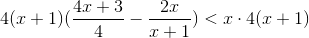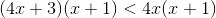2) Simplify: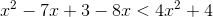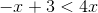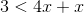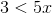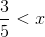3) For standard notation, and the fact that inequalities can be read backwards:For interval notation: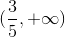4) Graph: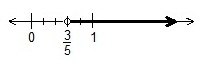### Example Question #1 : Solving Polynomial And Rational Inequalities

Solve and graph: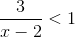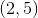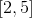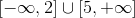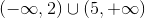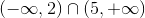Explanation:

Graph the rational expression,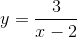1) Because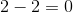and a divide byis undefined in the real number system, there is a vertical asymptote where.

2) As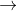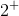,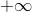, and as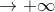,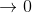.

3) As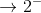,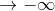, and as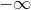,.

4) The funtion y is exists over the allowed x-intervals: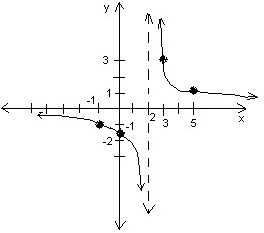One approach for solving the inequality:

For1) Determine where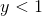over the x-values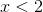or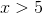.

2)for the intervals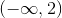or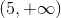.

3) Then the solution is.

Another approach for solving the inequality:

1) Writeas, then determine the x-values that causeto be true: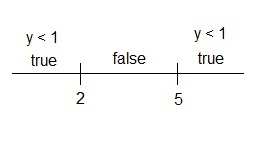2)is true foror.

3) Then the solution is.

### All Precalculus Resources